# Electrochemistry Class XII Solved NumericalsElectrochemistry Class XII Solved Numericals

Que 1. 0.05 M NaOH solutions offered resistance of 31.16 Ω in a conductivity cell at 298 K. If cell constant is 0.367 cm–1. Find out the molar conductivity of NaOH solution.
Ans 1. Given Molarity = 0.05 M
Cell Constant = 0.367 cm-1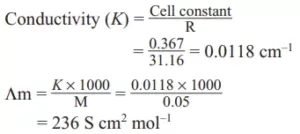Que 2.  Consider the following cell reaction:
2Fe(s) + O2 (g) + 4H+ (aq) → 2Fe2+ (aq) + 2H2O(l); E0 = 1.67V. At [Fe2+] = 10–3 M, p(O2) = 0.1 atm and pH = 3. What will be the cell potential at 250C?
Ans 2. Here n = 4, and [H+] = 10– 3 (as pH = 3)
Applying Nernst equation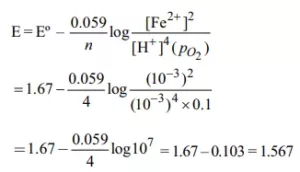Que 3. A salt solution of 0.30 N placed in a cell whose electrodes are 1.9 cm apart and 3.6 cm2 in area offers a resistance of 20 Ω. Calculate equivalent conductivity of solution.
Ans 3. Resistance (R) = 20 Ω
Length (l) = 1.9 cm a = 3.6 cm2 , Normality (N) = 0.30 N
Cell constant = l/a = 1.9/3.6 = 0.528 cm-1
Specific conductance (K) = Conductance × Cell constant = (1/R) × cell constant
= (1/20) × 0.528 = 0.0264 S cm–1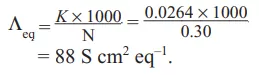Electrochemistry Class XII Solved Numericals

Que 4. The electrode potential E (Zn2+/Zn) of a zinc electrode at 25°C with an aqueous solution of 0.1 M ZnSO4 is.
Ans 4.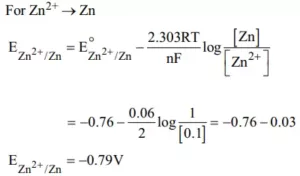Que 5. The molar conductivities of CH3COOH at 25°C at the concentration of 0.1 M and 0.001 M are 5.20 and 49.2 cm2 mol–1 respectively. Calculate the degree of dissociation of CH3COOH at these concentrations. (Λm (CH3COOH) = 390.7 S cm2 mol–1).
Ans 5.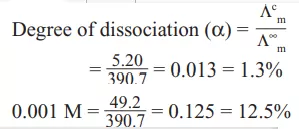Que 6. If 0.01 M solution of an electrolyte has a resistance of 40 ohms in a cell having a cell constant of 0.4 cm–1, then find its molar conductance in ohm–1 cm2 mol–1.
Ans 6. Molarity = 0.01 M
Resistance = 40 ohm
Cell constant l/A = 0.4cm-1.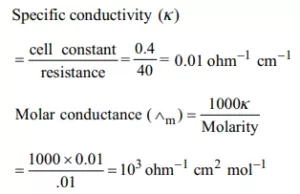Electrochemistry Class XII Solved Numericals

Que 7. If Λm of HCl, NaCl, and CH3COONa is 425, 128, and 96 Ω–1 cm2 mol–1 respectively, calculate the value of Λm  for acetic acid.
Ans 7. Λm CH3COOH = Λm CH3COONa + Λm HCl – Λm NaCl
= 96.0 + 425.0 – 128.0 = 393.0 Ω–1 cm2 mol–1.

Que 8.

 Electrolyte KCl KNO3 HCl NaOAc NaCl Λ∞ (Scm2 mol–1) 149.9 145 426.2 91 126.5

Calculate ΛHOAc using appropriate molar conductance of the electrolytes listed above at infinite dilution in H2O at 25°C.
Ans 8. ΛHcl  = 426.2 ………………(1)
ΛAcONa = 91.0 …………………………….(2)
ΛNaCl = 126.5 ………………………….….(3)
ΛHOAc = (1) + (2) + (3)
= [426.2 + 91.0 + 126.5] = 390.7 Scm2 mol–1

Que 9. How many hours does it take to reduce 3 moles of Fe3+ to Fe2+ with the 2.0-ampere current? (F= 96500 C mol–1).
Ans 9. Fe3+ +  e → Fe2+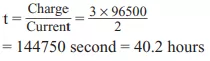Electrochemistry Class XII Solved Numericals

Que 10. At 25°C, the molar conductance at infinite dilution for the strong electrolytes NaOH, NaCl, and BaCl2 is 248 × 10–4, 126 × 10–4 and 280 × 10–4 Sm2mol–1 respectively. Find Λ0m Ba(OH)2 in S m2mol–1.
Ans 10.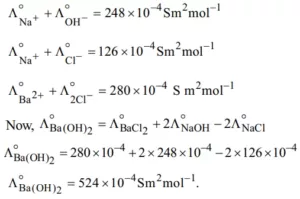Que 11. How many grams of silver can be plated out on a serving tray by electrolysis of a solution containing silver in +1 oxidation state for a period of 8.0 hours at a current of 8.46 amperes? What is the area of the tray if the thickness of the silver plating is 0.00254 cm? The density of silver is 10.5 g/cm3.
Ans 11.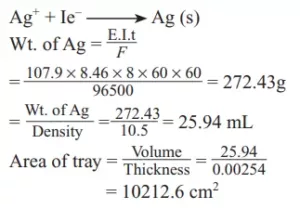Que 12. Find the number of coulombs required to reduce 12.3 g of nitrobenzene to aniline.
Ans 12.  As per the equation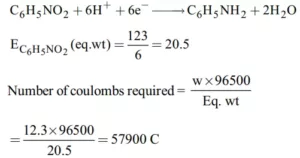Electrochemistry Class XII Solved Numericals

Que 13. Calculate the quantity of electricity required to reduce 6.15 g of nitrobenzene to aniline if the current efficiency is 68%. If potential drops across the cell is 7.0 volts. Calculate the energy consumed in the process.
Ans 13. C6H5NO2 + 6H+ + 6e → C6H5NH2 + 2H2O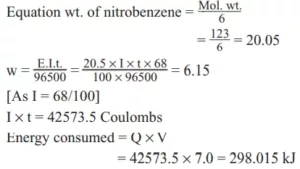Que 14. Calculate the cell potential of the given cell at 25°C. (R = 8.31 J K–1 mol–1, F = 96500 C mol–1).
Ni (s)|Ni2+(0.01 M) || Cu2+ (0.1 M)|Cu(s) Given: E0Cu2+/Cu = + 0.34 V E0 (Ni2+/Ni) = – 0.25 V.
Ans 14.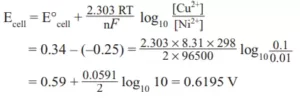Que 15. Calculate the cell potential for the cell containing 0.10 M Ag+ and 4.0 M Cu2+ at 298 K. Given E0Ag+ /Ag = 0.80 V, E0Cu2+/Cu = 0.34 V
Ans 15. Cu (s)|Cu2+ (4.0 M) || Ag+ (0.1 M)|Ag (s) Here n = 2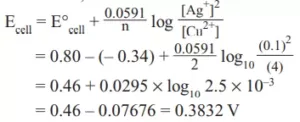Electrochemistry Class XII Solved Numericals